# Just About Everything You Need to Know for the ACT Math Section

We can never know what exactly is going to be on the ACT Math section, but each test draws from a similar bank of concepts and terms. This is great news, because it means if you know you tend to struggle on the ACT math section, you can look over this comprehensive list of ACT topics to boost your ACT score.

Some terms and concepts appear on every test, and others appear on every other test or every fourth test. Below is a list of the most common ACT math concepts and how often they tend to appear!

## Which Topics are Most Important to Know for the ACT Math Section?

We know this giant list of ACT math concepts seems scary — but don’t worry, we’ll go over each of them in this article. If you see one on the list and you feel like you don’t understand it as well as you could, scroll down for an explanation!

## Must Know ACT Math Concepts

These concepts will appear on the ACT Math section. They make up the core material on the exam and students preparing for the test should know these topics first before approaching more

### Fractions

Fractions can be expressed in two different ways:

• As a ratio of two integers: 2/3. This is the form used when a fraction is equal to a value less than 1 or when when a fraction is larger than 1 but does not simplify to a whole number: 5/2. The latter case is known as an improper fraction, or a fraction where the numerator (top half of the fraction) is greater than the denominator (bottom half of the fraction).
• Improper fractions can also be written as a mixture of whole numbers and fractions. For example, 5/2 can also be written as 2 1/2. Since these are a mixture of two different things, these are referred to as mixed numbers.

There are four different ways that fractions can interact: addition, subtraction, multiplication, and division.

For the first two (addition and subtraction), each fraction needs to have the same denominator, known as a common denominator. Once the fractions have the same denominator, they can be added or subtracted as follows:

• Add: Add the numerator and keep the denominator (bottom half of the fraction) the same
• Subtract: Subtract the numerator of the second fraction from the first fraction and keep the denominator the same
• Multiply: To multiply fractions, multiply the numerators of the fractions together, and also multiply the denominators of the fraction together
• Divide: To divide fractions by each other, it’s easiest to turn division into multiplication. Dividing by a fraction is the same as multiplying by its reciprocal (swap the numerator and the denominator: what was on top will now be on bottom, and vice versa). For example, to divide something by 12, that’s the same as doubling it, or multiplying by 2/1!

### Average

The average of a data set is also known as the arithmetic mean.

The mean is equal to the sum of all the data points divided by the number of data points in the set

### How It Will Appear on the Test

• Mean-Median Comparison: Many ACT questions about the mean of a data set will ask students to compare the mean and the median: which is larger/smaller or are they the same? Data sets with numbers that are close to one another in value will have similar or identical means and medians. Data sets with more variation (or that are skewed in one direction or another) will have more distance between the mean and median.
• Individual Data Point Changes: Questions may also ask: how does increasing or decreasing one of the data points in the set change our mean? What data points would need to be added to get the mean to a particular value?
• Calculating Mean from a Table or Graph: Can you calculate the mean of a data set from its histogram? A table of values?

### Probability

The probability of an event occurring is equal to the number of successful outcomes (outcomes that fulfill the pre-set criteria) divided by the number of potential outcomes.

For example, to calculate the probability of drawing a red skittle from a bag of skittles, a student will need two pieces of information:

1. How many red skittles are there in one bag
2. How many total skittles (regardless of color) are there in one bag

The probability of drawing a red skittles is then equal to: # of red skittles / # of total skittles.

The key for probability questions is whether the question is asking us to add or multiply probabilities together.

• Adding Probabilities: Add probabilities when there are multiple different possibilities that will fulfill the probability criteria. So add each discrete probability together to get the total probability.

How does a student know if there are multiple different possibilities that will fulfill the criteria? In the problem above, it is not specified that a particular red skittle is needed (e.g. the heaviest or the largest). Any red skittle will do, so the total probability adds together the individual probability of getting each individual red skittle and that gives the probability of getting a red skittle.

The main key word for an added probability question is: OR. If scenario A or B could be a success, then the probability of being successful is = the probability of A + the probability of B (as long as these two things are not mutually exclusive. In other words, that there is no overlap between these possible outcomes).

• Multiplying Probabilities: Multiply probabilities when there are multiple different criteria/steps that each need to be fulfilled in a sequence (or multi-step process). Each step in the process has to be a success, and the probability has to account for the possibility that any given step might have been unsuccessful. This requires multiplication of probabilities rather than addition.

For example, using the skittles setup from above: if a question asks about the probability of drawing two consecutive red skittles, then that will require calculating the probabilities of each individual draw, and then multiplying them together. Another way to imagine multiplying probabilities is as a tree of possible outcomes.

If the odds of drawing a red skittle are ⅔, then on the first draw, there needs to be two branches: the branch where the first draw is successful (which will happen 2 out of 3 times) and the branch where the first draw is unsuccessful (which will happen 1 out of 3 times). Those are the odds of the first draw.

One must then repeat the process for my second draw. Since one may have been successful or unsuccessful on the first draw, the probability has to account for both possibilities when the final probability is calculated. The odds of ending up on one of the final four outcomes branches (for example red, red) = the odds of going down the first branch x the odds of going down the second branch.

It is sometimes possible to get a mixture of multiplied and added probabilities. This occurs in a multi-step process that has multiple possible successful outcomes.

For instance, if a question asked what the possibility of drawing two skittles of the same color (red + red or blue + blue) would be. In that case, the tree still looks the same. But instead of focusing on the odds of one outcome branch, one must instead add up the odds of the two outcome branches that fulfill the goal.

This concept would be written out as this equation: (1/9+ 4/9= 5/9)
It’s worth noting that in the tree above, the probabilities are treated as independent. That is, the odds of one draw do not affect the odds of another draw. However, this is not always going to be the case. It will depend on whether or not I have outcomes that are mutually exclusive.

To understand how mutual exclusivity comes into play, and to look at our above concepts in action, here is question #46 from the December 2020 ACT: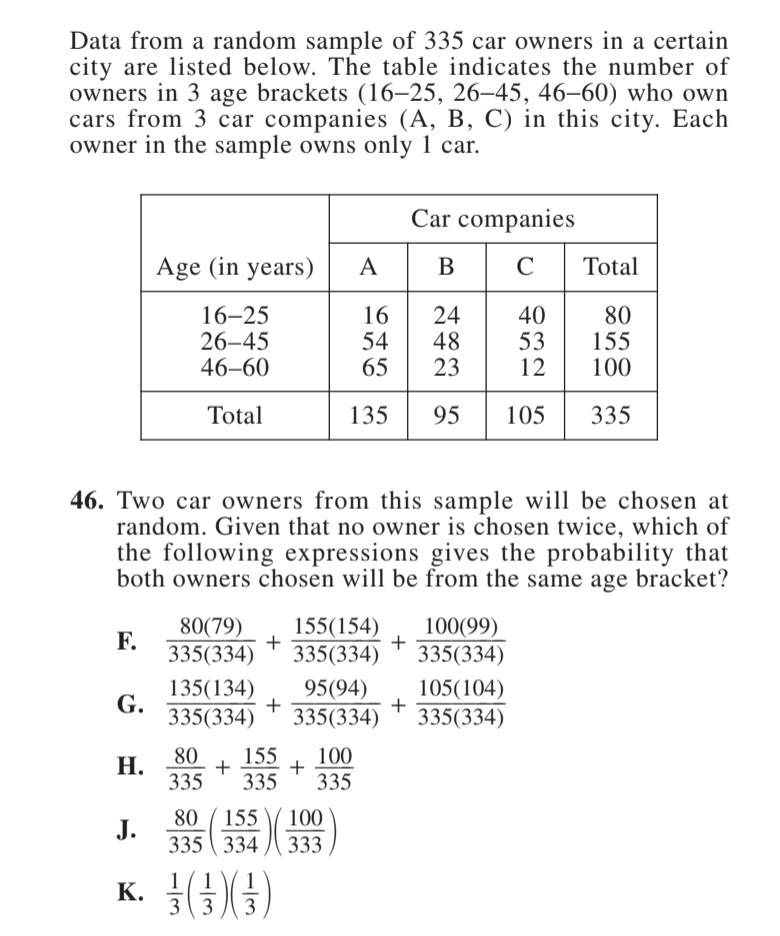The question wants to know the odds that two car owners drawn from our overall sample will be from the same age bracket. It can be any age bracket, so the question can be restated as: “what are the odds that the first car owner AND the second car owner picked will both be from the 16-25 age bracket OR the 26-45 age bracket OR the 46-60 age bracket?”

The question is asking to find two different car owners. This means that after selecting the first driver, they are no longer going to be in the pool of possibilities that will be selected from: they were selected in the first step, so they cannot be picked in the second step. Therefore, both the numerator and denominator of the probability fraction by 1 (to account for removing one successful possibility from our pool or possibilities). There is one fewer possible successful option and one fewer possible option overall.

So, for this problem, it is necessary to determine what the probability is that one will pick two car owners from each age bracket.

There are 80 16-25 year olds in the sample out of a possible 335 drivers. That means the odds of drawing a 16-25 year old are 80/335. The 2nd draw requires a different person from the same age bracket. So, there are 79 remaining drivers that could be successfully drawn from the 16-25 age bracket. There are also 334 total drivers now remaining. That means the odds of drawing the 2nd person from that age bracket are 79/334.

Both of those steps need to happen to fulfill the question’s criteria. Since step 1 AND step 2 need to be a success, the total probability of drawing two drivers from the 16-25 year old age bracket is (80/335)(79/334).

The process is then repeated for the other two age groups, since each separate age group can fulfill the specified criteria.

There are 155 people in the 26-45 age bracket. The odds of drawing someone on the first go are 155/335 and then 154/335 on the 2nd, since one still cannot draw the same person twice. That makes the odds of succeeding on both the 1st AND 2nd draw (155/335)(154/334).

Similarly, there are 100 people in the 46-60 age bracket. The first and second steps odd are 100/335 and 99/334, and the total probability is their product: (100/335)(99/334).

The odds of picking two drivers from each of the three different age brackets has been calculated. Each of those possibilities represents a successful outcome, and so the answer is the sum of their probabilities:

That means that A is the correct answer!

#### Key Takeaways

• Multiple steps = multiplication
• Mutual Exclusivity = Reduce the numerator and denominator

### 4. Percents

Percents express what the numerator of a fraction would be if the denominator was 100 (80% = 80/100). 100 is the standard base, and whenever a student is thinking about percentage questions, they must always want to think about that idea of the base.

It is possible to have percentages of numbers other than 100.

75% of 160 = 120. Put another way 75% = 120/160. In this case 160 is the base, and that determines the %.

The most common mistake ACT students make on percentage questions is to accidentally change the base of the percentage fraction, oftentimes without knowing it. ACT percentage questions will always contain wrong answers based off of this mistake.

For example, here is Problem #59 from the June 2020 ACT.

This problem includes two different price markdowns, and so there is a chance to make two different base errors here. Adding together the two discounts 10% + 30% leads to answer E. However, these percentages were discounts off of two different prices (and therefore also two different bases). Trying to add them together is like adding fractions with different denominators: it simply cannot be done.

Instead, a student must use the inverse percentage, so that everything stays in terms of the original price. Saying that something is 20% off of the original price is the same thing as saying it is 80% of the original price.

In the problem above, the sales price of a jacket is 10% off of the original price. This means that the sale price = 90% of the original price, or that the Sale Price = 0.9(Original Price).

The clearance price is 30% off of the sale price. This means that the clearance price = 70% of the sale price, or that Clearance Price = 0.7(Sale Price).

Combining these two equations together, shows that Clearance Price = 0.7(0.9)(Original Price) = 0.63(Original Price).

The clearance price is 63% of the original price, meaning that it is 37% off (100-63) of the original price.

The other wrong answers in this question come from getting caught up with the original percentages given. For example, it is easy to think that since the sales price is 10% off of the original price, then the original price is just 110% of the sales price.

However, that’s flipping the base of the percentage. The store took 10% of the original price, not 10% of the sales price. So adding 10% to the sales price is likely to underestimate the original change. This is why it’s good to simply keep things in terms of the original price Sale Price = 0.9(Original Price).

You are much less likely to make an easy error if you keep things in the units/terms of the original numbers in the problem.

## 5. Exponents

The main test with exponents is to know when to perform the core three math operations to exponents:

### Three Main Exponent Operations

Exponents are added together when we those exponents share the same base number and that number is multiplied by itself

x2 • x5 = x5 + 2 = 7 = x7

• Subtract:

Exponents are added together when we those exponents share the same base number and that number is multiplied by itselfThe number in the denominator is subtracted from the number in the numerator.

$$\frac{x^{5}}{x^{2}} = x^{5 – 2\ = \ 3} = x^{3}$$

• Multiply

Exponents are multiplied by one another when a base number and its exponent are raised to another power (when an exponent-base pair has an exponent).

(x5)3 = x5 • 3 = 15 = x15

There are also three special exponents that students are expected to know. Instead of using operators, these are types of exponents. Students must know the different ways to express these exponents, and how that can then affect the operations listed above:

### Special Exponents

• Negative Exponents

Negative exponents signify that a number is being taken to the inverse of the given exponent.The exponent expression gets moved from the numerator to the denominator.

$$x^{- 4} = \frac{1}{x^{4}}$$

A key takeaway is that a negative exponent doesn’t lead to negative numbers. The negative here is about inversion, not positive/negative.

• Fractional Exponents

Fractional Exponents are another way to express roots.The root that students are generally most familiar with is the square root. Square roots are thought to be the default when the root operator is used. But this could also be written as$$\sqrt{}$$if a student wanted to be clear that it’s a square root (or root 2). This makes then makes it easier to understand the rule that:

$$x^{\frac{1}{2}} = \sqrt{x}$$ The denominator becomes the root power.

$$x^{\frac{2}{3} = \sqrt{x^{2}}}$$ If there is a number other than one in the numerator, that exponent stays with the base number under the radical, even when we switch to the root expression.

It’s important to remember: Fractional exponents do not lead to the base switching from numerator to denominator (as happens with negative exponents)

• Zeroed Exponent

Any number taken to the 0 power equals 1.

x0= 1 30 = 1 ( − 13)0 = 1

## 6. Linear Equations/Slope

### Key Skills

#### Calculate Slope from Two Points

If given two points on a line, a student should be able to calculate the slope of that line.

The phrase often used to teach this is “rise over run”. Calculate the change in y values and divide it by the change in x values.

$$\frac{y{}_{2} – y_{1}}{x_{2} – x_{1}} = m$$ It’s assumed that the a line moves from left to right when calculating slope, so the y2value should be the y-value of the right-most point, and the x2value should be the x-value of the right-most point. The other two values then come from the other point.

#### Isolate Y (Create y = mx + b)

Sometimes a linear equation will be given in a form other than the classic y = mx + b setup. For these questions, y is treated like any other variable: simply isolate it on the left hand side of the equation.

5X + 5Y = 35 becomes X + Y = 5 (divide whole equation by 5) and then

Y =  − X + 5 (−X from both sides)

This is a good reminder that whenever anything shows up on the math section that looks unfamiliar, try out some algebra and see if it can be made to look more familiar. Take the unfamiliar and make it look familiar.

#### Find Intercepts

The intercepts of a linear equation are where the line hits the x-axis and the y-axis. These are found by setting the opposing variable to zero: equations hit the y-axis/y-intercept when x = 0, equations hit the x-axis/x-intercept when y=0.

For the y-intercept, it’ll be as simple as plugging in 0 for x and then seeing what the output of the equation is. Finding the x-intercept generally requires a little more algebra, as a student works to isolate x.

### Key Information for Linear Equations

#### Perpendicular Slope

When two lines are perpendicular, their slopes are negative reciprocals of each other. That means we can take either slope and find the opposing slope by switching its sign and inverting it between the numerator/denominator.

If there are two lines, m and n, and line m has a linear equation y = 4x + 6, and line n is perpendicular to line m, then it must have a slope of: $$- \frac{1}{4}$$.

#### “Real Solutions”

“Real solution” questions about linear equations will generally start with two linear equations. What matters for these questions are the slopes and intercepts of the given pair of equations. Based on the number of solutions, the following things will be true about the slopes and intercepts of the two lines:

Real solution questions will either have students calculate the number of solutions for a given pair of equations, or it will state a number of solutions and ask students to create an equation that either satisfies this number of solutions (which one CAN be true) or which cannot satisfy that number of solutions (which one CANNOT be true).

Other keywords to look at for are:

Parallel: Parallel lines have no solutions

Intersecting: Intersecting lines have one solution.

Overlapping: Overlapping lines have infinite solutions.

This is a good reminder that another way to think about solutions with linear equations is as “points of intersection”. The solutions to pairs of equations are where those lines intersect on the xy-plane.

## 7. Solving Equations

### Basic Skills

#### Distribute.

Distributing involves applying a leading coefficient or sign to all of the terms within a pair of parentheses or other grouping of numbers/variables. For example, when a -5 is put in front of the binomial (x + b), it affects both of those terms, even though when it is written out ( − 5(x+b)) the coefficient is located next to the x variable and is separated from the b constant.

This means that when a student “distributes”  − 5(x+b) =  − 5x  − b. It’s important to note that not only the number is distributed, but also the sign. If both the sign on the coefficient and the sign in between the binomial terms are positive, then no signs will change on the terms. If the signs of the coefficient and between the binomial terms are either different or both negative, then a sign change will occur. As soon as a student sees a negative sign or a minus in between binomial terms, they should double check that they’ve distributed the signs correctly.

#### Combine like terms.

If there are two or more similar terms on the same side of an equation, those terms can be combined. “Like” or “similar” terms means terms with the same exponent and the same variable. This is why a student can combine 3x + 5x but not 3x + 5x2.

For example, if an equation looks like: 3x + 17 + 2x  − 5= 3x. On the left side of the equation there are two constant terms (terms that are just whole numbers) and two x terms (same variable and same exponent): these pairs can each be combined to simplify the equation.

This is how 3x + 17 + 2x  − 5= 3x becomes 5x  + 12  = 3x

### Special Skills

#### Creating Equations from Word Problems

Some ACT word problems are much easier to solve with equations, even though the initial question might not provide an equation. Here is an example from the July 2020 ACTClearly there are two things that the question wants students to keep track of: time and distance. The key information given by the equation are the two speeds, the directions (due west/east, meaning the drivers are moving horizontally), and the total distance traveled by the two drivers.

In this case, to make the math easier, it’s important to recognize that even though the question asks about the intersection of two different journeys, it’s not necessary to create two different equations. The two drivers will pass each other when they’ve driven a total of 240 miles between them.

The equation that can be created is: 240 = 57x + 68x or (after combining like terms)

240 = 135x. This is because the drivers cover 135 miles (combined) each hour.

$$x\ = \ \frac{240}{125} = 1.92$$ This is the number of hours it will take for the two drivers to pass each other/drive the 240 miles.

Since the question wants a time in minutes, the hours need to be converted: $$1.92\ hours\ \bullet \frac{60\ minutes}{\text{hour}} = 115.2$$ One hour and 55 minutes after the initial departure time = 3:55pm. The answer is D.

## 8. Picking Numbers

Some questions on the ACT can be made easier by plugging in real numbers in place of variables. Picking numbers can give a student a more intuitive understanding of the problem, instead of keeping things very theoretical.

When choosing numbers for a problem, it can be helpful to try and identify: what is the core mathematical concept the problem is trying to touch on?

For example, let’s look at Problem #41 from the December 2020 ACT.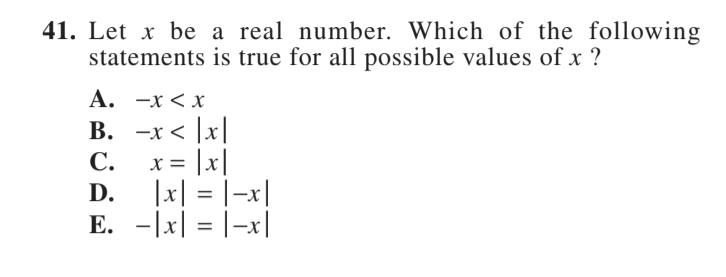This problem can be thought about conceptually (meaning leaving the variables in rather than testing actual numbers), but that can be hard to understand sometimes. To make it easier, a student can test out some values and see if they can figure out which statement is true for all values of x. Looking at the possible answers to the question, it is easy to notice that there are four different answers that involve the absolute value.

Since absolute value equations change negatives to positive, it is likely worthwhile to choose a negative number to test. For example, a student can pick the number -2. Once they’ve got the test number, they can start testing to see if each answer is true for the test value (if it’s false for the test value, then it is not true for all values like the question stipulates.)

It’s important to note that test values can prove that a statement is not true, but they cannot necessarily prove that a statement is true.

− x < x  − (−2)  <  − 2 2  <  − 2

This statement is not true for all values.

− x < |−x|  − (−2) < |−(−2)| 2 < |2| 2  < 2

This statement is not true for all values

x = |x|  − 2 = |−2|  − 2 = 2

This statement is not true for all values.

|x| = |−x| |−2| = |−(−2)| |−2| = |2| 2 = 2

This statement is true for at least our test value (not necessarily all values)

− |x| = |−x|  − |−2| = |−(−2)|  − |−2| = |2|  − 2  = 2

This statement is not true for all values.

The test value shows that A, B, C, and E are not true for all values, which means that D must be the answer. Again, it’s worth noting that just because D worked at our test value, it didn’t necessarily mean it had to be true for all values. If a student had chosen positive two (2) instead of negative two (-2), some of the statements above would have been true. This is why it was important to choose a negative value, so it was clear how the absolute values affected the outcome.

## 9. Ratio

Ratios tell the proportional quantity of one thing to another. Ratios are often expressed either with a colon – the ratio of kids to adults is 6:1 (read six to one, meaning there are six kids for each 1 adult) – or like a fraction – the ratio of kids to adults is $$\frac{6}{1}$$.

It’s important to remember that even though ratios can look like fractions, they are not the same thing. Fractions express a part of a whole. Ratios show proportional quantities. Put another way, fractions relate part to whole. Ratios relate whole quantity to whole quantity.

One great example of this is that ratios can be flipped, but fractions cannot. The fraction $$\frac{1}{2}$$is not the same as $$\frac{2}{1}$$. But if a student is looking at a ratio, then the positions of the numbers don’t matter, as long as the labels are kept consistent.

In the kids example, it was given that the ratio of kids to adults is 6:1. This could also be stated as: the ratio of adults to kids is 1:6. As long as both the label and the number are flipped, the meaning stays the same.

This also works if when a ratio is expressed like a fraction:

$$\frac{\text{Kids}}{\text{Adults}} = \frac{6}{1}$$ and $$\frac{\text{Adults}}{\text{Kids}} = \frac{1}{6}$$.

Now, even though ratios express different meanings than fractions, there are certain properties of fractions that can be used on ratios expressed like fractions. For example, if one takes the ratio $$\frac{\text{Kids}}{\text{Adults}} = \frac{6}{1}$$and multiplies both sides by the variable adults, then this leads to the equation Kids = 6 x Adults. So if a student would like to find out the number of kids in a given situation, they can simply multiply the number of adults by 6.

So a student can work with these ratio-fractions as if they’re normal fractions, as long as they keep in mind that they don’t mean the same thing as a normal fraction.

Ratio questions will often ask a student to relate multiple ratios to each either. A student might be given the names of three people, as well as ratios of those individuals’ incomes or heights, for example. They could also be given ratios that ask you to combine information about a larger group, and then information about parts of that larger group. Or they might be given two different versions of the same ratio – simplified and unsimplified – and be asked to calculate one from the other.

For example, here is Question #46 from the April 2021 ACT: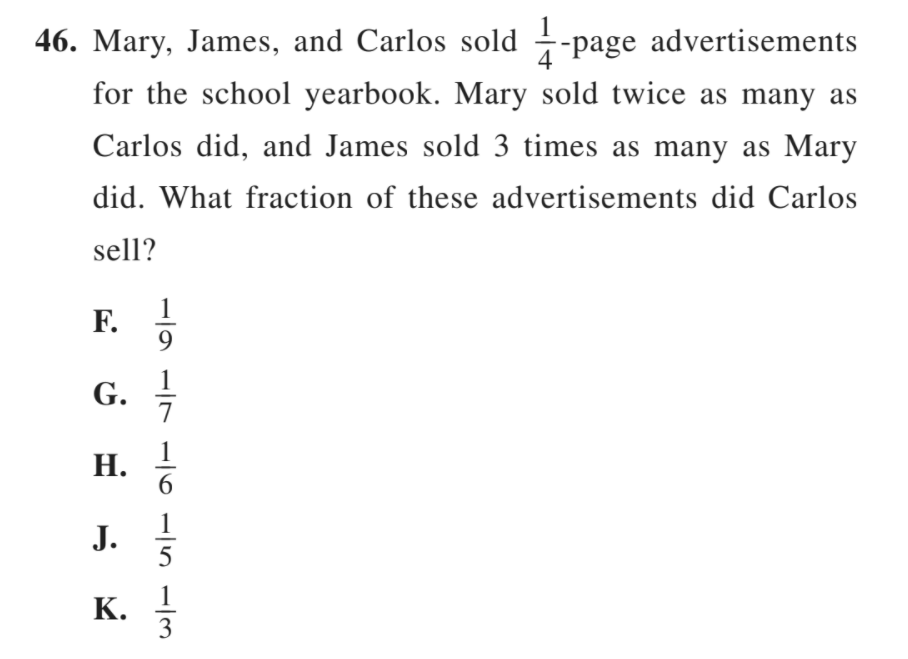Here the ratios are about advertisement sales. Note that they include the $$\frac{1}{4}$$page number at the beginning just to be confusing. The fact that these advertisements are that size does not affect the answer or how a student should approach the question.

Mary sold twice as many advertisements as Carlos. $$\frac{\text{Mary\ Sales}}{\text{Carlos\ Sales}} = \frac{2}{1}$$

James sold three times as many advertisements as Mary. $$\frac{\text{James\ \ Sales}}{\text{Mary\ \ Sales}} = \frac{3}{1}$$

Since Mary made two sales for every sale that Carlos made, that means that James made 6 sales for every sale that Carlos made. One can find this by rearranging the equations from above to look like: Mary Sales = 2  •  Carlos Sales and James Sales = 3  •  Mary Sales.

Therefore James Sales = 3 • (2•Carlos Sales) = 6 Carlos Sales

James sold 6 Carlos Sales worth, Mary Sold 2 Carlos Sales worth, and Carlos sold 1 Carlos Sales worth. Therefore, there were 9 Carlos Sales worth of total sales, and Carlos sold 1 out of the 9. So the answer is F.

There are four crucial skills a student needs to have when it comes to quadratic equations: to factor, foil, zero parentheses, and graph parabolas.

To start, it’s worth remembering what a typical quadratic equation looks like:

ax2 + bx + c

There are three terms: a quadratic (squared) term, a linear term, and a constant.

• Factor

Quadratics are often expressed in the above format, but the ax2 + bx + c form isn’t necessarily super intuitive. Oftentimes, it’s helpful to factor a quadratic equation to make it easier to interpret. Factoring a quadratic means the same thing as factoring a normal number: looking for things that can be multiplied together to get the original number/expression.

To factor the number 27, one can either factor it by expressing it as 27 x 1, or as 9 x 3. The idea is the exact same with quadratics, but now there are both whole numbers and variables to work with.

Quadratic equations will often factor into two binomials (an expression with two terms that are either added or subtracted from one another). Binomials look like: (x + a) or (xb).

When looking to factor ax2 + bx + c, one is first going to look at the aand c terms. That’s because if the quadratic equation factors cleanly, these two numbers will be the product of only two things.

ax2comes from the two x terms multiplied together. c comes from the two constant terms (a and b) multiplied together. Since these two terms are only the product of two numbers, it gives a student the most direct information about what the factors might be.

One usually starts with the c term, since it’s the most basic. C is just the product of two constants. So C is factored like any other integer.

The example quadratic here will be:

x2 + 5x + 6

c = 6. The factor pairs of 6 are; 2 and 3; 1 and 6. The binomial factors need to include one of these two pairs.

a = 1. The leading coefficient on the quadratic term is 1, so there are no coefficients in front of the variables in the binomials. The middle term products are also not scaled by anything.

Since the sign on c is positive, the two constants inside the binomials need to have the same sign. Since the middle term b is positive, it’s clear that the constant terms will also be positive.

This equation requires two binomials with no coefficients on the x, which multiply to 6 and add up to 5. Therefore, the factor pair of the constants is 3 and 2.

So factored, the above equation would look like: (x+3)(x+2)

• FOIL

FOIL stands for: First, Outside, Inside, and Last. It’s a way to remember how to multiply out two binomials.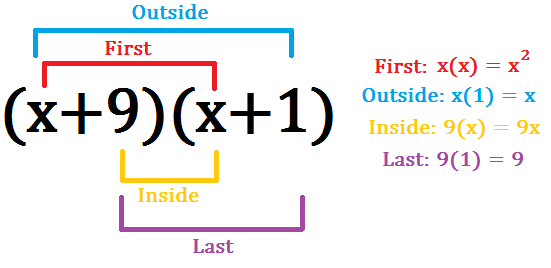The first two terms of each binomial are multiplied together. Then the numbers on the outside of the expression as a whole, followed by the two numbers on the inside of the expression as a whole. Finally, multiply together the last two numbers from each binomial.

These four things are then added together to tell one what the total product of these two binomials is equal to. The First and Last terms normally stand on their own, since they are usually the products of two variables and two constants, respectively. In comparison, the Outside and Inside terms can usually be added or subtracted together, since they will usually both be the product of a variable and a constant.

Check out the explanation in #55 on conjugates below to see why they create quadratic equations with just two terms when FOILed!

• Set parenthesis equal to zero

Part of the use of factoring quadratic equations into binomials with variables and constants is that it can make key information about the equation much easier to see. For example, when equations are factored into binomials such as (x−5)(x+3), the x-intercepts of the graph are much easier to find than when the equation is expressed as x2 − 2x − 15.

To find the x-intercepts, simply set each parenthetical pair = 0. Another way of thinking of it is to ask: what number would need to be substituted for x to set the parentheses to 0. The answer should always have the opposite sign from the sign in between the two binomial terms. If there is a minus sign, then the answer should be positive. If there is a plus sign, then the answer will be negative.

• Graph parabolas

Students should be able to look at a quadratic equation and immediately know what the graph of that equation should look like.

For example, the equation: y =  − (x+1)2 + 2

The main things to look at when trying to graph a parabola are:

• If the leading coefficient is positive then the parabola should be upward facing and the parabola will have a minimum point

If the leading coefficient is negative then the parabola should be downward facing and the parabola will have a maximum point

In the example above, the leading coefficient is -1, meaning that the parabola is downward facing and has a maximum point.

##### Intercepts

The intercepts show where the parabola will hit its maximum or minimum point.

• The x-value of this minimum point is equal to the solution to the parabola’s binomial. In the sample equation above this solution = -1
• The y-value of the minimum point is the value of y at the solution point. For the equation above y=  − (1−1)2 + 2 = 2
• Combining these two things shows that the maximum point for the parabola should be at (-1,2)

Not all equations will have only one quadratic in them. For example, a problem could ask about the graph of: (x−3)2(x+2)3

There are two key things to look at here:

• Exponent: The exponent says what the equation will do at the given intercept.
• If the exponent is linear (raised to the first power), then the function will cross the x-axis at that intercept
• If the exponent is quadratic (raised to the second power), then the function will “bounce” off the x-axis at that intercept
• If the exponent is cubic (raised to the third power), then the function will “slide” at the x-axis (it will cross, but not in a linear fashion. Steeper further from the intercept and shallower close to the intercept)
• Intercept: The factors of the equation show how many x-intercepts the graph should have.
• There will be a unique intercept for every unique value of x that could set the equation to 0. This is usually found by looking at the constants in the binomials and seeing if there are any numbers repeated in the parenthesis and which have the same sign. If the sign and constant are repeated, then this does not create an additional intercept.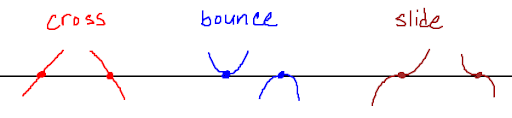• “Real Solutions” with Quadratic Equations

When a question presents a quadratic equation and asks about “real solutions” or simply “solutions”, there is a two step process to finding the answer the question is looking for.

Step 1: Try to Factor

See if the quadratic equation factors cleanly. If the quadratic equation can be factor into two binomials with whole numbers, then that will always be the quickest and easiest way to find “solutions” to that equation. Because this is the easier way to solve quadratic equations, questions that are designed for this method will often come earlier in the math sections. They also will often be the problems that mention just “solutions” rather than “real solutions”. Non-real solutions tend to show up more during the second step.

Step 2: Look at the Discriminant

$$\frac{- b \pm}{2a}$$ For these questions, it’s necessary to use the quadratic equation. The most important portion of that equation is called “the discriminant”, which refers to the portion of the equation that is underneath the square root: b2 − 4ac.

The discriminant determines if there is a negative, positive, or zero number underneath the square root. This then determines how many “real” answers there are to the equation.

A negative number under a square root means that there are only non-real answers (answers that include i (. Zero under the square root means that the equation simplest to just $$\frac{- b}{2a}$$, which since a and b are constants, which will lead to just one answer. And finally a positive under the square root leads to a positive number, which will then be added and subtracted from  − b, which then creates two answers.

Just like with linear equations, questions about real answers for quadratic equations will either ask a student to find how many real answers there are for a given equation (unlike with linear equations, there is just one quadratic equation, since quadratics can have multiple solutions on their own) or they will ask a student to create an equation that either CAN or CANNOT be true if the equation is to have a given number of real solutions.

## 11. Area/Perimeter of basic shapes

On the ACT, students may be asked to calculate the areas of certain common shapes, including triangles, rectangles, and circles. The test will provide students with these formulas, but it’s good to be comfortable with using them before the test, so that questions involving these concepts are just about automatic!

• Triangle

The area of a triangle is = $$\frac{1}{2}\text{bh}$$ where b is the length of the triangle’s base and h is the height of the triangle. For right triangles the height will be equal to the vertical side coming up from the triangle. For non-right triangles, the height is equal to the distance from the base of the triangle to its opposite corner.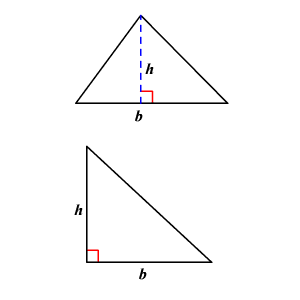• Rectangle

The area of a rectangle is equal to the base times the height.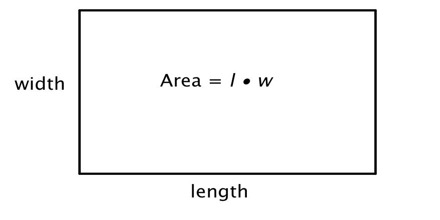• Circle

The area of a circle is = πr2where r = radius of the circle.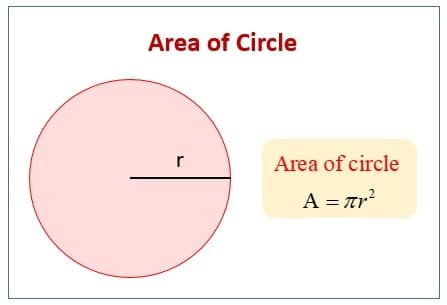With circles, it’s worth noting that some questions might ask students to use a portion of the area of a circle. This means students just need to use the internal angle measurement of the sector in question to convert the total circle area into the sector area.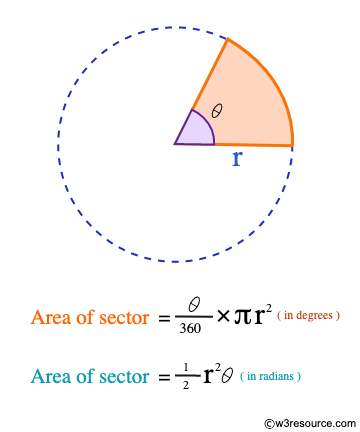## 12. Negatives

The easiest simple mistake to make on an ACT math section is to make a sign error. Whether it’s addition, subtraction, division, or multiplication, negatives make it easy to switch a sign (or to forget to switch a sign) and lose points on a question that a student would otherwise have gotten right.It’s important for students to be comfortable with how negatives are affected or not affected by the normal math operators: this starts with addition and subtraction.

### Negative Operators

Adding negative numbers is just like adding positive numbers. The sum of two negative numbers has the same magnitude as the sum of the same two positive numbers.

a + b = c  − a + (−b) =  − c

The notation used here is worth paying attention to. Since  − b is technically being “added” to  − a in the second equation, it has to be put in parenthesis, since two operator signs cannot go next to each other. This can be helpful in preventing mistakes, as whenever students see a parenthesis between an operator and a negative number, that’s a great time to slow down and double check work.

• Subtract

Subtracting numbers is a bit different than adding numbers, since subtracting a negative number means taking away something less than zero, which actually translates to adding something. The phrase that many people are familiar with is: two negatives make a positive.

− 5 − (−3)=  − 5 + 3 =  − 2.

• Multiply

Multiplication can either be like addition or subtraction depending on how many negative numbers are being multiplied. If there is an odd number of negative numbers being multiplied by any number of even numbers, then the total product will be negative.

− 5 • 2 •  − 4 • 3 •  − 2 =  − 240

Any two pairs of negative numbers will cancel out their negatives and produce a positive, and any positive numbers added will just keep the product positive. Therefore, if there is an even number of negative numbers being multiplied together with any number of even numbers, then the total product will be positive.

− 2 • 4 • 1 •  − 3= 24

• Divide

Division is the same as multiplication, but where the pairs of negative numbers have to be in the numerator and denominator to cancel out signs with each other. If both numbers in a fraction are negative, then their signs will divide out and the final product will be positive. If only one of the numbers (in either the numerator or the denominator) is negative, then the final product will be negative.

### Negative Tricks

• Distribution

The most common way to make a negative sign mistake on any given problem is by not distributing the negative properly when multiple terms are multiplied by the same negative. For example:

− 5(a+4) =  − 5a  − 20 Since the -5 is right next to the a, it’s easy (on a timed test when a student is rushing) to think that the -5 only distributes to the variable a and to leave the + 4 alone. But since +4 is inside the parentheses, it needs to be multiplied by the -5 when it is distributed.

## 13. SOHCAHTOA

SOHCAHTOA is a pneumonic device used to remind students of the three trigonometric functions and their equivalents on a right triangle. Problems utilizing SOHCAHTOA will often have students solve for a missing side length on a triangle, or occasionally find a formula to express a side length (where there may only be variables or a mixture of numbers and variables, but where a final number cannot be solved for).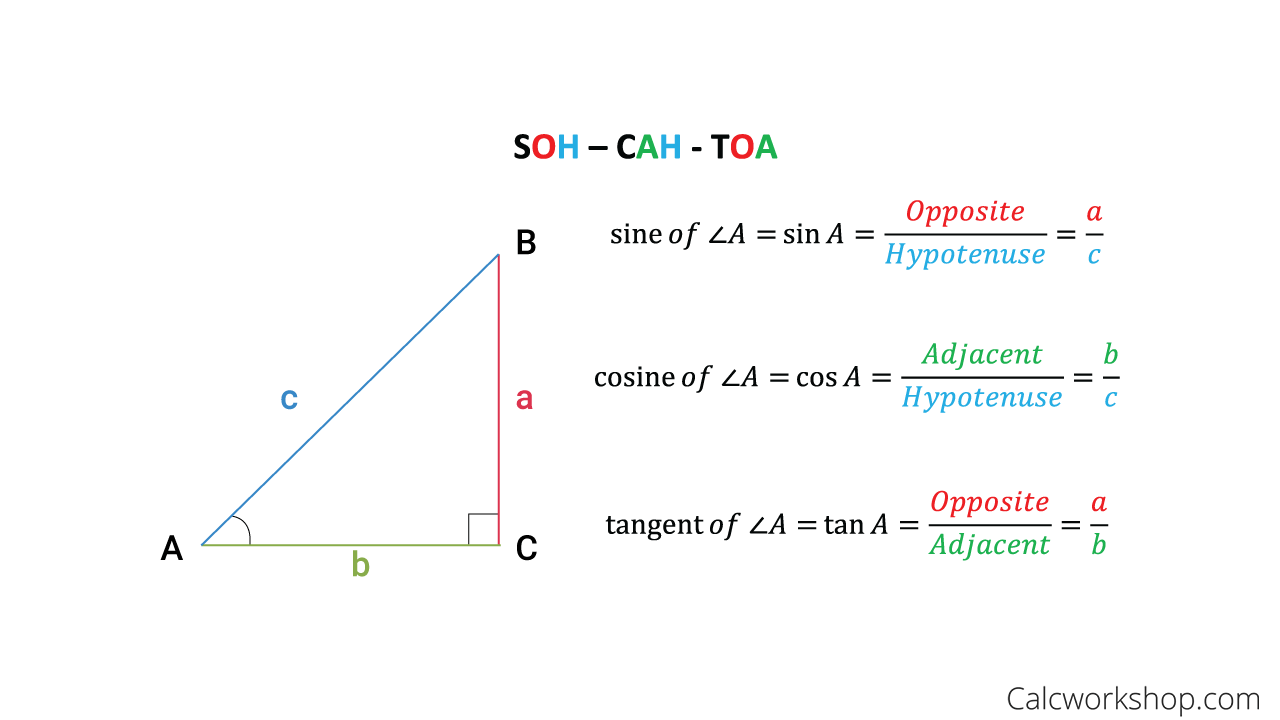One tip for remembering the letters themselves is that each of the side labels (adjacent, opposite, and hypotenuse) is used twice. There are two Os, two As, and two Hs. Remember either that Tan doesn’t use hypotenuse or that Sin and Cos do, and the rest of the relationships will fall into order.

It’s also worth remembering that these identities are true for both acute angles of a right triangle. And since the labels of “adjacent” and “opposite” are relative to which side is being used, there is some overlap between these identities.

### Sin and Cos Overlap

The Sine of the 1st acute angle of a triangle is equal to the Cos of the other acute angle, and vice versa. In our examples above, sinA = cosB and sinB = cosA.

### Word Problems

SOHCAHTOA is most common on problems that look like our image above, where there is a triangle on its own and the problem will look to have students solve for a given angle or side. But SOHCAHTOA can also be used to solve word problems.

The triangle may already be drawn in (like the example below) or the question may simply describe a situation that creates a right triangle. The key thing to remember is that: right triangle + trig functions = SOHCAHTOA

The example below is from the July 2019 ACT. The problem references the following information provided above the question itself: the maximum altitude during Tour C is 1,000 feet.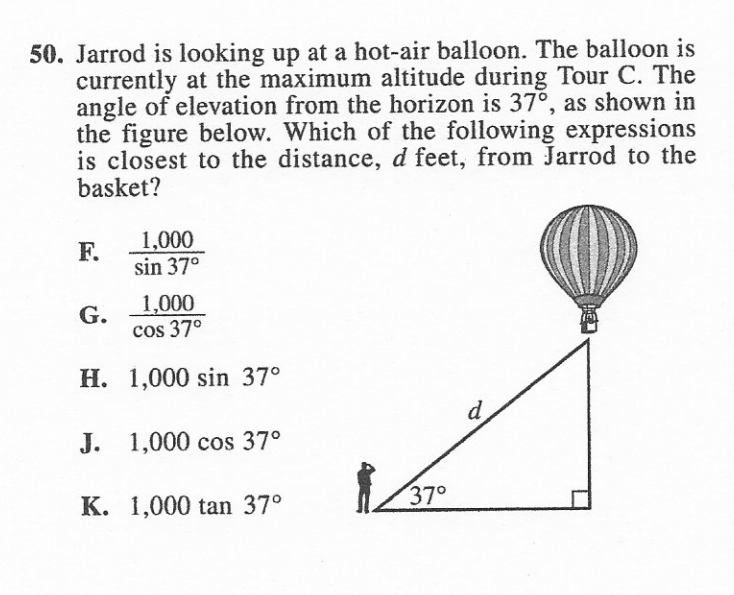The angle 37 is used in all of the answer choices, and so the answer will involve SOHCAHTOA, the 37-degree angle, and the height of 1,000 feet.

The question asks about the length of the hypotenuse of the triangle and the question gives the length of the side opposite the angle. Therefore, this is a situation where SOH ($$sin\ = \ \frac{\text{Opposite}}{\text{Hypotenuse}})$$will be relevant.

$$sin37\ = \ \frac{1,000}{c}$$ A little algebra then leads to: $$c\ = \frac{1,000}{sin37}$$. F is our answer.

# Extremely Likely (> 80% chance):

## 14. Function shifts

### Vertical Shifts

Vertical function shifts are highly intuitive. They occur from either a coefficient placed in front of the variable(s) in the function or because of a constant term added to the function equation.

Sample Function: y = 2(x+1)2 − 5

Here the function is shifted down by 5 units, and is multiplied by a factor of 2. The directions of these changes are intuitive, as they are the same size and direction as the numbers in the equation.

### Horizontal Shifts

Horizontal shifts can be a bit more counterintuitive. For example, in the sample equation above the x variable has a + 1 next to it. Following the same rule as for vertical shifts, it would make sense that the x-intercept would then move one unit in the positive direction on the x-axis (to the right).

However, it’s actually the opposite. (X+1) means a horizontal shift one unit to the left. This is also true for leading coefficients. (2X) does not expand a graph in the horizontal direction, it shrinks it.

## 15. Average sum trick

The average sum trick simply refers to the idea that if a question gives the average of a data set and the number of data points in that data set, then that also allows a student to calculate the sum of all of the data points in the set.

This is equal to: average  • # of data points

This is important to know for questions about adding or subtracting data points.

For example:

5 tests, average is 80. After the 6th test, the average is 82. What was the 6th test score?

Average Sum = 80  • 5 = 400

The new average is going to be equal to: (the old sum + the new number)/the updated # of data points

$$82\ = \ \frac{400\ + \ 6th\ score}{6}$$ (82•6) − 400 = 6th score The 6th score was a 92.

This works the same for subtracting a data point. The only difference is that the denominator is reduced by 1 instead of increased by 1, and the removed test score is subtracted rather than added to the original sum.

## 16. MPH

Miles per hour is a way to express speed: distance covered over a time interval. Questions using MPH often involve some kind of unit conversion. This can be a conversion of distance units (miles to kilometers) or time units (hours to minutes).

MPH also shows up in word problems very often, where a student might need to create an equation with a variable for “hours” (the variable choice is based off of the units that speed is originally given in or that the student is trying to solve towards).

## 17. Median

The median is the middle number of a data set when the data set is arranged in numerical order. Unlike the mean, it will always be one of the actual data points in the set itself. The set can be arranged as ascending or descending order: the median will still always be in the middle.

When a student wants to find the middle/median number in a data set, they must take the number of values in a data set (n = 23 for example) and divide it by two.

### Median of Odd-Numbered Data Sets

In the example listed above, n= 23/2 = 11.5. Because there is an odd number of values in the data set, dividing this number by two results in a nonwhole number. For an odd-numbered data set, we will take this partial number and round it up, in this example to 12. This tells us that the middle number, in other words, the median, is the 12th number in the data set.

### Median of Even-Numbered Data Sets

For even-numbered data sets, there is not one number in the middle of the data set. Instead, there are two middle values, and the median is the average (mean) of those two numbers. This is why if there was a sample of 24 data points (instead of the 23 from above), the same operation from above leads to a whole number: 24/2 = 12. The 12th data point is the first of the two median numbers. To get the 2nd number, round up to the next whole number, which tells us that the 13th data point is the second of the two median numbers. The median is then the average of the 12th and 13th numbers.

Remember, for this trick the product of the equations is not equal to the value of the median itself, but merely its “place in line” in the data set. It says where to look, but not what the median is.

### How It Appears on the Test

Median questions are similar to average/mean questions. Students will normally be asked to do one of two things. Either they will be asked to calculate the median of a given data set (displayed through a table or graph) or they will be asked to describe how a change in the data set will or won’t change the median (or what change(s) would need to be made to the data set to lead to a certain change in the median).

Radicals (also known as “roots”) are the opposite of exponents. The most common radical that students are familiar with is the square root or root-2, which is why the default radical symbol is also the square root symbol: . A square root basically says: to get the number under the square root, what number would need to be multiplied by itself to get that number?

Roots above a square root will have a subscript next to the root to show what “degree” the root is taken to. Again, this is the same as exponents where Xmeans x cubed. In the same way, $$\sqrt{x}$$ is the cube root of x. The first expression says what happens when x is multiplied by itself 3 times. The second expression asks what would need to be multiplied by itself three times to get x.

#### Cancel Out

Because radicals and exponents express opposite operations, they can cancel out with each other under certain circumstances. For example, a square root and an exponent of 2 will cancel out with each other.

= x The same thing is true for any evenly balanced roots and exponents

$$\sqrt{x^{3}} = x$$

#### Multiplication

Two separate square roots multiplied by each other are equivalent to the square root of the products of those numbers

#### Simplification

The rule above can also be used to help simplify square roots. Instead of combining square roots though, it will involve separating them.

For example, take the square root of 48. This is equal to the product of the roots of its factors: sqrt(48) = sqrt(4⋅12). The square root of 12 is not a whole number, but the square root of 4 is.

Therefore, the expression can be simplified as 2sqrt(12). There are still only whole numbers here, but now if there was a or a 2 in the denominator below , it would be clear that these would cancel out and that the expression could be simplified further.

Whenever a root appears with a whole number underneath it, students should ask themselves: “is there a way to factor this number such that I can simplify the expression?”

This also works when there are variables under the root.

#### Division

Division with roots is the opposite of multiplication. The ratio of the square roots of two numbers $$\frac{}{}$$ is equal to the square root of the ratio of those two numbers.

#### Fractional Exponents

As mentioned in the exponents section, roots can be expressed as fractional exponents. If a number has a fractional exponent, then the denominator (bottom half) of that fractional exponent is the root that that number is being taken to.

$$x^{\frac{1}{2}} =$$ This doesn’t affect or change exponents in the denominator. Those remain in the normal exponent spot, even when the fractional exponent is converted to the root form:

$$x^{\frac{3}{2}} =$$

## 19. System of Equations

System of Equations involves taking a series (usually two) of equations and finding their “solution”, meaning variable values that make all equations in the series true. Another way to think of this is to imagine graphing those equations, and a system of equations problems asking students to find the intersection points between the given equations.

### Two Methods for Systems of Equations: Elimination and Substitution

#### Substitution

The substitution method of system of equations involves isolating a variable in one of the equations, and then plugging in that expression for the variable into the other equation.

For example, if a question gives the following system of equations:

2x + 3y  = 2

x − 2y = 8

Then to substitute, a student must choose one equation and one variable to isolate. Isolating the x variable in the bottom equation gives x  = 2y + 8. That expression is then substituted in for the x variable in the top equation: 2(2y+8) + 3y = 2. This then leads to 7y=  − 14 or y =  − 2.

#### Elimination

Just like with any algebraic equation of coure, one can either work with variables individually or with the equation as a whole. With systems of equations, questions are generally looking for solutions that make both equations true. Since that means the equations must be true statements, the two equations can be multiplied by, divided by, subtracted from, or added to each other, just like any other true statements.

The reason the word “elimination” is used to refer to this is because the most common way to make the two equations in a system of equations interact directly is to subtract one equation from another. Specifically, a student should look to subtract one equation from another such that one of the two variables in those equations is eliminated.

To do this, the variable should have the same coefficient but opposite signs in each equation. This may already be true when the equations are first stated in the problem, or one equation may need to be manipulated to make this work.

In the example above, neither variable has the same coefficient but opposite signs, so a little work would need to be done.

The bottom equation could be multiplied by -2, which would lead to the x variables having same coefficients and opposite signs. A student could also look to eliminate the y variable, but this would require either multiplying one equation by a non-whole number or manipulating both equations. With the ACT, speed is always of the essence, so students should try to choose the quickest/most efficient path!

Therefore, the bottom equation is multiplied by -2:  − 2x + 4y =  − 16

The two equations are then added together. Technically either side can be added together, but it’s easiest to add the variable sides and constant sides together.

7y = -16 is our sum, or y =  − 2. Same answer as our previous method, but just done a little differently.

### Deciding Between Substitution and Elimination

Students should do what they’re most comfortable and confident with.

That being said, there are some general guidelines for choosing between these two methods, if a student is comfortable with either approach.

#### Look at the Numbers in the Equation

First, look at the numbers in the equations. As the following questions:

• Are there any shared coefficients and opposite signs in front of the variables?

If yes, that means no work is needed and elimination will be the easiest route.

• Are the constants and coefficients factors of each other? Would they divide into each other evenly or cleanly?

If yes, then it could be easy to manipulate the equation and utilize elimination

If no, then it could be difficult to eliminate numbers easily. substitution may be best.

#### Look at the Question

What is the question asking for?

• Does it want the value of a single variable or of multiple variables or the product/sum of two variables?

If it is asking for a single variable, which cannot be easily reached through elimination, then substitution is often helpful.

If it is asking for the product or sum of multiple variables,  especially if that product or sum can be reached through elimination or by leaving an equation intact.

#### Word Problems

Sometimes problems will involve systems of equations, but the equations will not be directly given by the question. Instead, the problem might be given as a word problem, but where the information presented would clearly lead to the creation of a pair of equations. With these equations there should be two variables, which can be used to calculate two different things: time and distance, for example.

Here is an example question from the April 2021 ACT.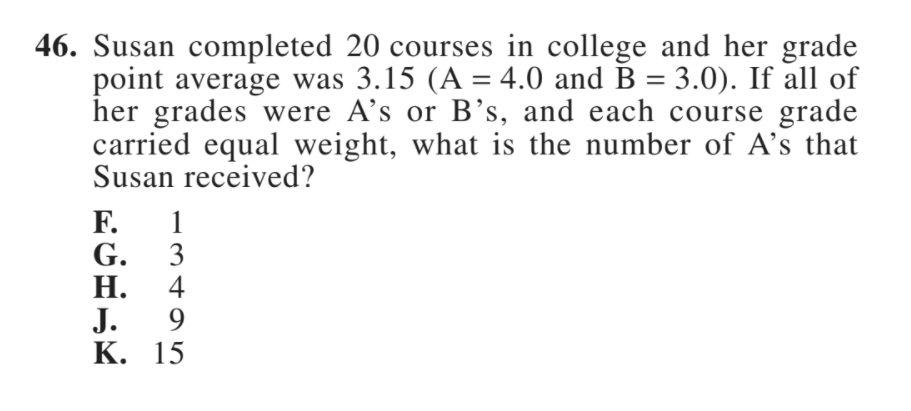20 = A + B where A and B are the number of courses Susan received an A or B in, respectively

$$3.15\ = \ \frac{4A\ + 3B}{20}$$. Again, we can use either substitution or elimination here.

− 3(20 = A + B) ⇒  − 60 =  − 3A − 3B

20 ($$3.15\ = \ \frac{4A\ + 3B}{20}$$) ⇒ 63 = 4A + 3B

3 = A Susan received 3 A’s

One important note: since the question asked for the value of A, B was the variable that the student cancelled out. If A had been cancelled out, then that would have added an extra (and unnecessary) step.

Cancel out or substitute in for the variable that you’re not solving for: this will leave just the variable you’re solving for in the equation.

## 20. Angle chasing

### How Many Degrees in ______ ?

#### How Many Degrees in a Line

There are 180 Degrees in a Line. This means that if there are two angles across a line, then the sum of those two angles will be 180 degrees. These are known as supplementary angles.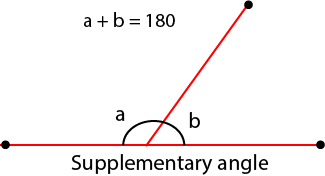#### How Many Degrees in Common Shapes

There are a number of common shapes that it is useful to know the number of degrees within.

• Triangle: 180 degrees
• Square: 360 degrees
• Circle: 360 degrees (sometimes also expressed as 2π radians)

#### How Many Degrees in Any Shape

While normally the common shapes listed above are all that students need to know. Occasionally there are weird shapes in angle problems, and knowing the number of degrees within those shapes may be crucial for answering that question.

Thankfully, there is a simple equation for knowing the number of degrees in any polygon

Number of Degrees in a Polygon = 180 (# of sides of the polygon−2)

For example, this is why a triangle has 180 degrees:180 (3−1) = 180  • 1 = 180

#### Vertical/Opposite Angles

Vertical Angles are angles opposite each other at the intersection of two different lines. The lines can have any slope (they do not have to hit at a right angle or anything special). As long as they are straight lines, opposite/vertical angles will be congruent (have the same measure).

In the illustration below, angle a = angle b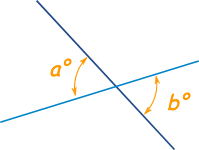### Angles at Intersections with Parallel Lines

When a line intersects a pair of parallel lines, there are a number of set relationships between the angles created by those lines. These are useful to know, because they can be massive time savers. You can save a full minute on an angle question just by going beyond just the supplementary angles rule (which can be tedious to work out with many lines or many shapes).

Depending on which pair of angles are being compared, the angles will either be congruent (equal) or supplementary (adding to 180 degrees), as follows: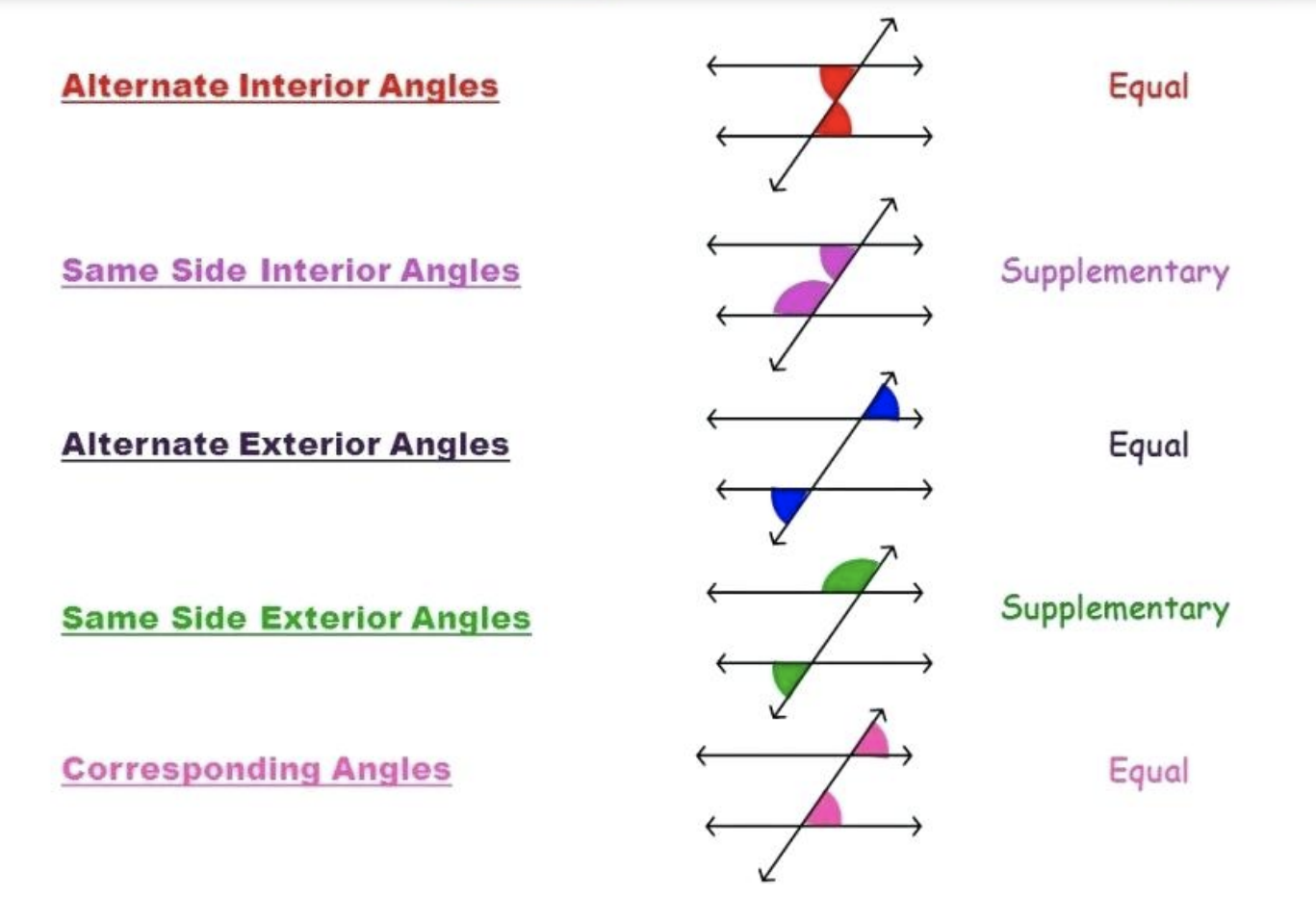## 21. Time

#### Time Conversions

Be able to convert between different units of time. The most common ones being between hours and minutes and between minutes and seconds.

#### Time Variables

Some equation questions might have variables that stand in for time, especially as an exponent. The question may then ask for how to maintain the same equation using a different variable for time.

For example, taking the equation T = 100(1.05)twhere t is the time in hours. If a question wanted this converted to minutes, but without changing the actual equation, then there will need to be compensation for the unit change.

Going from hours to minutes means that the exponent would become larger if there was no compensation. As a result, the new variable will need to be divided by 60 (as this is the number of minutes in an hour).

Therefore, the new equation would look like: $$T = 100(1.05)^{\frac{m}{60}}$$where m is the number of minutes.

The key for keeping equations consistent while changing exponent variables is that if you’re subbing in a bigger variable, then it will need to be divided by something, and if you’re subbing in a smaller variable, then it will need to be multiplied by something.

## 22. Pythagorean Theorem

This is one of the most fundamental concepts in math, and as a result it is something that will be applicable on every single ACT. The pythagorean theorem applies to right triangles and states that the square of the hypotenuse is equal to the sum of the squares of the other sides.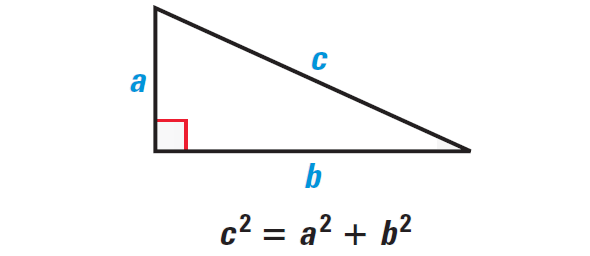A question may simply show a triangle directly and ask for either the side lengths or a calculation that requires knowing all of the side lengths (such as perimeter). This may also be usable in a word problem.

Be on the lookout for if a word problem explicitly states that something happens at a 90-degree angle or if there is a perpendicular line or tangent line. These all lead to 90 degree angles and mean that a right triangle may be created, and so the pythagorean theorem is valid.

## 23. Apply formula

Applying formula problems will provide a student with a particular formula/equation, and will then ask a student to properly plug in numbers into that equation to calculate something. This will often be done in the context of a word problem that describes the real-world application of the equation. For these equations the main tricks are to:

• Clearly identify the dependent and independent variables. The independent variables are what is being changed in the equation (in the cause/effect chain, they are the cause). The dependent variables are the outputs of the equation. THey are the result of the change made.
• Be sure to remain consistent with units. The original formula might be given in hours, but then the number that has to be plugged in is in minutes. Remember that the original formula is the guide, and that everything should be kept consistent with that guide.

## 24. Composite function

Composite functions are where one function is put inside of another. This can look intimidating at first, but these problems are quite simple once a student gets the hang of it.

### Inside Out

It’s easiest to think of composite functions as a priority list or an order of operations. Work from the inside to the outside.

Here is an example from the June 2020 ACT: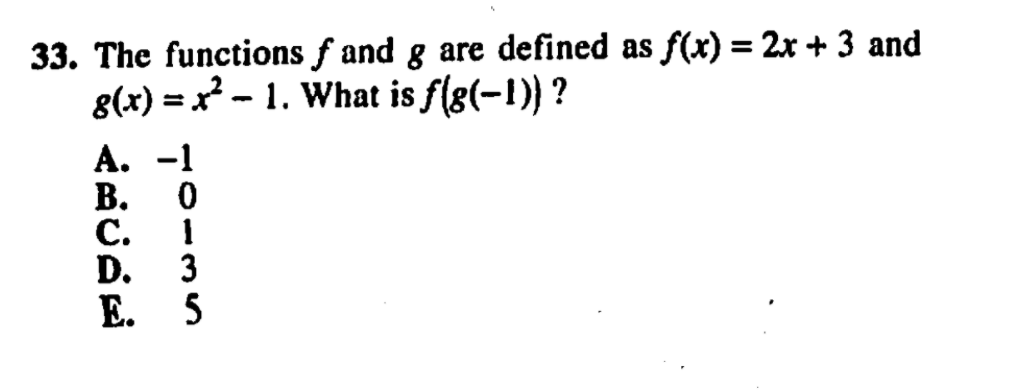Working from the inside out. g(−1) = (−1)2 − 1  = 1 − 1 = 0. Since g(−1) = 0, g(−1) can be replaced with 0, as if it was a variable that we had solved for and could now plug into an equation.

Now the next layer: f(0) = 2(0)  + 3 = 3. The answer is 3!

## 25. Matrices

A matrix is a series of rows and columns used to display numbers. ACT questions about matrices will generally be about performing fundamental math operations such as addition, subtraction, or multiplication between matrices.

### Is It Possible?

A key note is that not all matrices can be added, subtracted, or multiplied.

• Two matrices can only be added or subtracted if they have the same dimensions
• Two matrices can only be multiplied if the number of columns in the first matrix is equal to the number of rows in the second matrix

Adding and subtracting matrices is quite simple. Since the matrices have to have the same dimensions, each number has a “twin” that shares the same space in the opposite matrix.

Matrix addition means adding each pair of numbers in the same places in their respective matrices. Subtraction means subtracting pairs.

The resulting matrix will have the same dimensions as each of the original matrices.

### Multiplying

Multiplying matrices is a bit more complicated. Matrix multiplication is used to find the dot product. This is sometimes the term used on the ACT to imply matrix multiplication.

As stated above, matrices can only be multiplied if the number of columns in the first matrix is equal to the number of rows in the second matrix. The top left number in the dot product is equal to the sum of the numbers in the first row of the first matrix multiplied, in order, by the numbers in the first column of the second matrix.

The next number down in the dot product’s first column is equal to the numbers from the second row of the first matrix multiplied, in order, by the numbers in the first column of the second matrix.

This is then repeated until there are no more rows left in the first matrix. The process is then repeated again for the next column in the second matrix.

The final dot product will have the same number of rows as the first matrix and the same number of columns as the second matrix.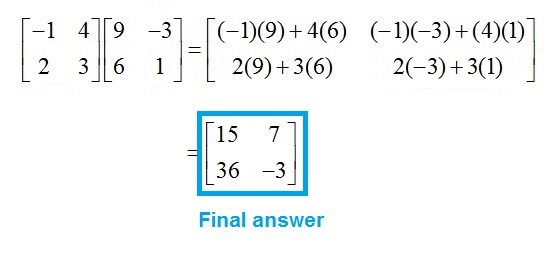## 26. Midpoint

Midpoint questions involve working with two ordered (x,y) pairs and finding their midpoint. This is done using the following equation:

$${(x}_{M},y_{M}) = (\frac{{x_{1}}_{} + x_{2}}{2},\frac{{y_{1}}_{} + y_{2}}{2})$$, where (xM, yM) is the midpoint and the x and y values on the right side of the equation are from the ordered pairs of that midpoint.

Occasionally these questions might give the midpoint and one of the ordered pairs, in which case a student must use normal algebra on the above equation to find the missing pair. Each variable will need to be dealt with separately for that algebra to work (it cannot be done simultaneously).

# Very Likely(> 50% chance):

## 27. Special Right Triangles

There are certain special triangles that students might be expected to know on the math section of the ACT. Like the formulas for the area of a circle or rectangle, these identities are normally presented to students at the beginning of the test, and so the challenge is knowing how to identify problems where these identities are relevant and how to use them to solve relevant problems.

The most common special triangles are: 45-45-90 and 30-60-90 triangles.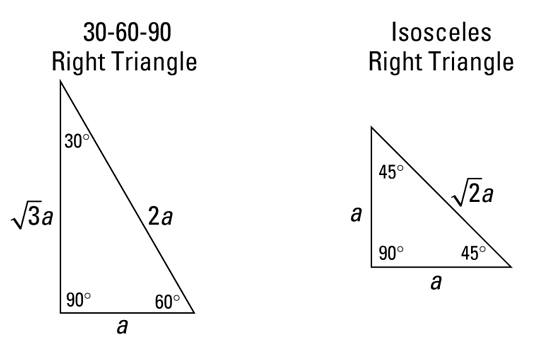Because these length and angle relationships are set, any time these angles appear or these side lengths appear, they can give us useful information for solving a problem. Problems involving these triangles often utilize words/drawings or the xy-plane. They are also often relevant on problems involving triangles and points of tangency. For example: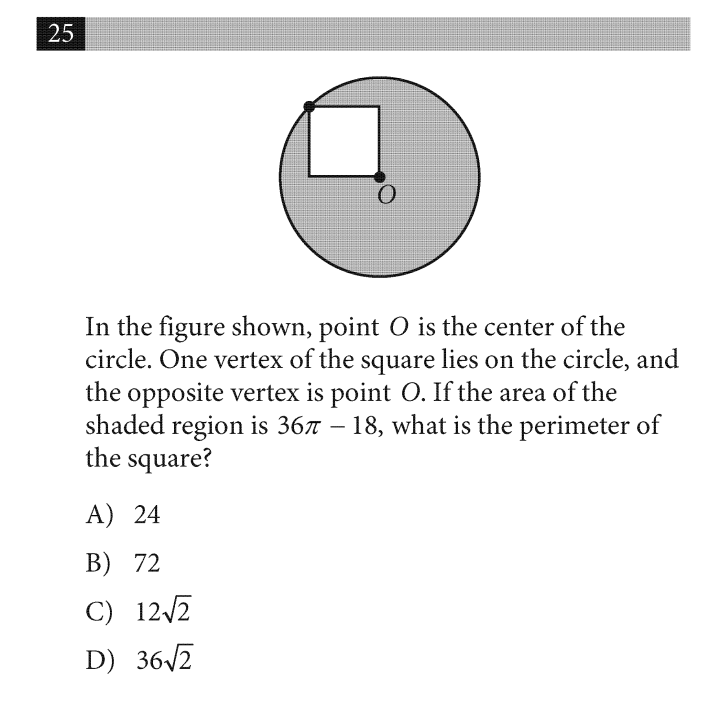Since the square touches both the center and the perimeter of the triangle, a line going from one corner of the square to the other corner will be equal to the radius of the circle. This straight line divides the square perfectly in half, which means that the angles on each side of the line are = 45 degrees. That means that this square can be defined as follows:

The question gives us the area of the shaded region, which equals 36π − 18. From the image, it’s clear that this is equal to the area of the circle minus the area of the square. In other words:

πr2 − s2 = 36π − 18 where r is the radius of the circle and s is the side lengths of the square. The triangle above shows that r = x. Plugging this into the formula above gives:

π(x)2 − x2  = 36π − 18

2x2π − x2 = 36π − 18

x2(2π−1)  = 18(2π−1)

x2 = 18 xx = 3

x was equal to the side length of the square and the question asks for the perimeter of the square. Therefore, the answer is: 4(3) = 12 and so C is the correct answer.

## 28. Absolute Value

|x(absolute value of x) is the magnitude (the non-negative value) of x, regardless of its original sign.

The concept of an absolute value revolves around negative and positive numbers. A positive number doesn’t change when placed in an absolute value, but a negative value does change: it becomes a positive. Therefore, if a problem involves an absolute value, it’s a good idea to pay attention to any negative numbers in the problem (or to use a negative number as a test value like in one of the above practice questions).

## 29. Multi Step conversion

Multi-step conversions will involve a mixture of ratios and/or equations. Questions will either go from a proportional ratio to an overall total, or they will ask for a conversion of one ratio to another (usually in the form of a unit conversion).

One of the most common versions of this question will involve mph and gas costs in miles/gallon.

## 71. Step Function

A step function is a function that includes a series of horizontal lines, which jump from one to the other at regular intervals.

• A step function graph will include open and closed dots. These open and closed dots pairs are where the function “jumps”
• The jumps will usually occur at whole number values of the x-axis

Here is an example question from the 2017 ACT: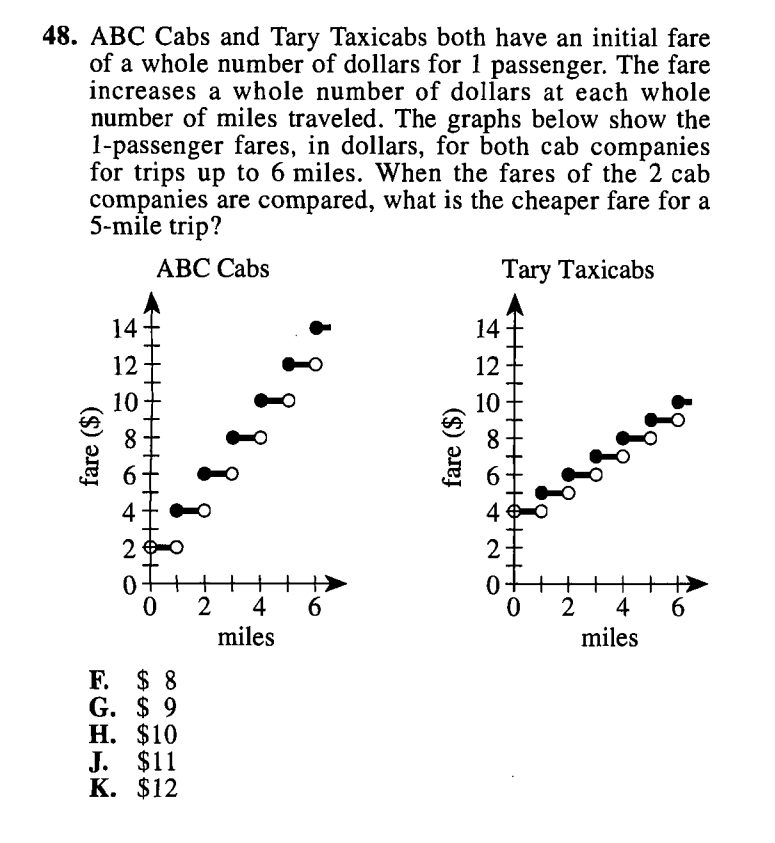For ABC cabs, the price of a taxi fare jumps $2 each time the fare reaches a new whole number value. This means for any distance of less than 1 whole mile, the fare is$2. As soon as 1 whole mile is reached, the price then jumps to $4. It stays at that price until 2 full miles are reached, and so on. The prices on the Y-axis are flat fares (they are not per mile). So to compare the price of a 5-mile fare for each company, a student must simply compare the y-values at x = 5 for both companies. For a 5-mile trip, ABC will charge$12 and Tary will charge $9. Therefore, the cheaper fare is$9.

## Bonus: General Math Problem Advice

### Things to Do With the Questions

#### Simplify

Sometimes an equation will be given in an unsimplified form. If a formula is given unsimplified, the first thing to do is to simplify it. The question is not written that way by accident, it’s done to make the problem seem more challenging or harder to solve. Once it’s been simplified, there is a good chance it will look more familiar or more manageable than it did in its original form.

#### Pull Out the Numbers

This is especially a good idea for word problems that seem confusing on first read. Remember that some words in word problems aren’t useful mathematically, and others are only useful when translated into something actually mathematical. Pull out numbers, variables, and any mathematical statements of fact (relative sizes, positive/negatives, sample sizes, etc).

#### Draw it Out

Especially true for graphing questions or word problems (especially if they seem to involve some kind of geometry).

### Things to Do With the Answers

If all of the answers have a certain denominator or include a special symbol (like a square root or negative), then that says the question revolves around that concept. Or that one needs to take the number/formula that is originally given and convert it to look more like the answer choices.

This should be done when a student doesn’t know how to approach a problem conceptually. It’s important to remember that it can take a fair amount of time, depending on how complex the problem/equation given is.

It’s never optimal to have to guess, but if one has to guess, then it’s best to give oneself the greatest odds possible of getting things right. When there are five possible answers, even eliminating two wrong answers can improve the odds of guessing correctly from ⅕ to ⅓.

It’s easiest to eliminate answers in batches. For example, if a student knows that the number has to be positive to be correct, then all of the negative answers can be eliminated. Same is true for if the answer needs to be even or to have a certain denominator.

## Boost Your ACT Math Score With SoFlo Tutors

Getting comfortable with these 71 concepts is a great start towards being successful on the ACT Math section. But there is always more to be done to be prepared, both for the math section and the English, Reading, and Science sections as well.

This can seem intimidating to do alone, and that’s why SoFlo Tutoring offers smart, reliable, and helpful tutoring services that are personalized for each individual student. High-scoring, expert tutors can help anyone to set goals, practice problems and concepts, and put themselves in the best possible position to be successful. Schedule a free consultation with SoFlo today for help on the SAT or ACT!

SAT/ACT Prep

SAT/ACT Prep